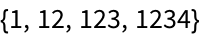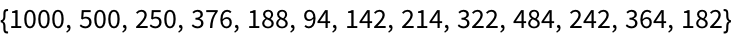47 Debugging Your Code
The Wolfram Language can sometimes tell as soon as you type something in that its likely to be wrongand then itll color it red.Something is obviously wrong when First is applied to the {} thats produced by Cases:
 In:=Out=Without values for a, b and c, the Wolfram Language just returns this unchanged:
 In:=Out=In:=Out=Use With to temporarily set m=4 to test a fragment of code:
 In:=Out=Make plots to see what the code is doing:
 In:=Out=In:=Out=Systematically check this up to m=10:
 In:=Out=Sometimes its not sufficient just to see the final result of a piece of code; you need to see whats going on inside as well. You can insert the function Echo anywhere to print intermediate results or values from your code.
Echo prints values, but does not interfere with the result:
 In:=1
2
3
 Out=In:=Out=Echo and Monitor just display things. If you want to actually capture intermediate results, you can do it using Sow and Reap.
Reap gives the final result together with a list of everything that was sown by Sow:
 In:=Out=This sows successive values of Length[#], and reaps the results:
 In:=Out=With[{x=value},expr] compute expr with x replaced by value Echo[expr] display and return the value of expr Monitor[expr,obj] continually display obj during a computation Sow[expr] sow the value of expr for subsequent reaping Reap[expr] collect values sowed while expr is being computed
47.1Correct the program Counts[StringTake[#, 2]&/@WordList[]] for counting possible first two letters in words. »
Sample expected output:
 Out[]=Answer & check your solution
47.2Use Sow and Reap to find intermediate values of #1 in Fold[10#1+#2&, {1, 2, 3, 4, 5}]»
Expected output:
 Out[]=Answer & check your solution
47.3Use Sow and Reap to get a list of all cases where #/2 is used in Nest[If[EvenQ[#], #/2, 3#+1]&, 1000, 20]»
Expected output:
 Out[]=Answer & check your solution
Can I cause a problem by just trying to run a piece of code?
Is there a most common type of bug in Wolfram Language code?
If I cant figure out whats going on, is it worth just trying random things?
Is there a way to do interactive step-by-step debugging in the Wolfram Language?
How can I tell whats wrong when a piece of graphics is pinked?
Its making a Gray codean ordering of integers so that only one binary digit changes at each step.